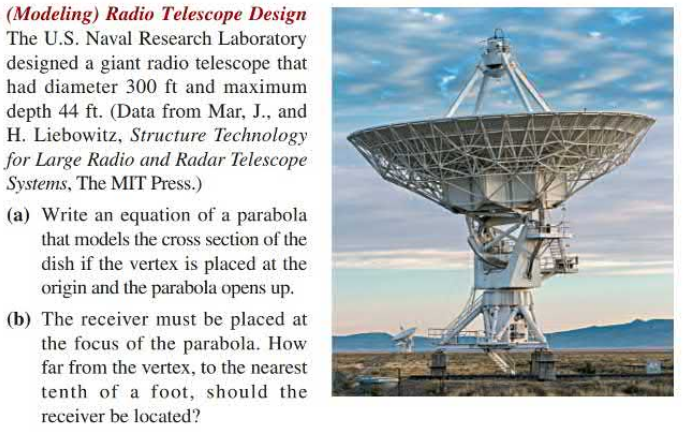### Still have math questions?

Algebra
Question(Modeling) Radio Telescope Design The U.S. Naval Research Laboratory designed a giant radio telescope that had diameter $$300 ft$$ and maximum depth $$44 ft$$ . (Data from Mar, J., and H. Liebowitz, Structure Technology for Large Radio and Radar Telescope Systems, The MIT Press.)

(a) Write an equation of a parabola that models the cross section of the dish if the vertex is placed at the origin and the parabola opens up.

(b) The receiver must be placed at the focus of the parabola. How far from the vertex, to the nearest tenth of a foot, should the receiver be located?

$$\left. \begin{array} { l l } { \text { (a) } y = \frac { 11 } { 5625 } x ^ { 2 } } & { \text { (b) } 127.8 ft } \end{array} \right.$$